# Thermodynamic, part 2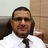- Assist.Prof of Laser Physics & Photonics en Ibb University, Yemen + Jazan University, KSA
2 de Sep de 2016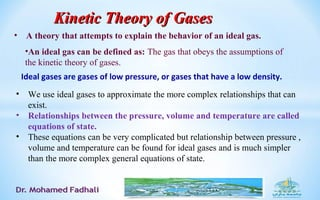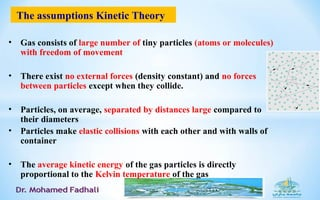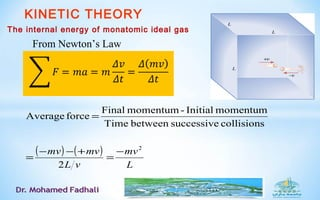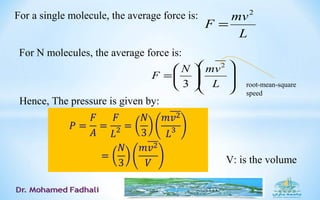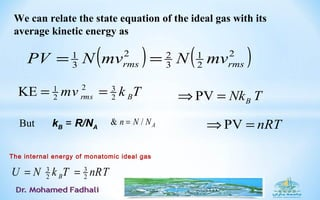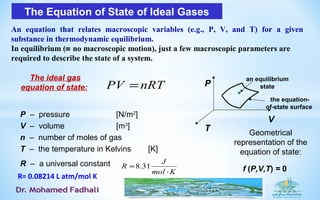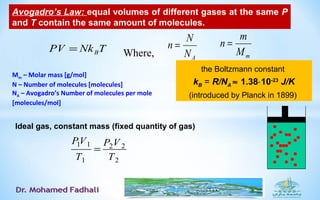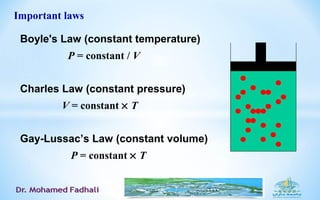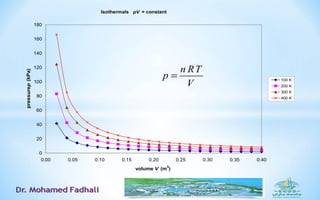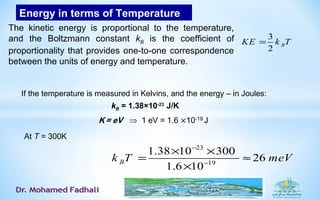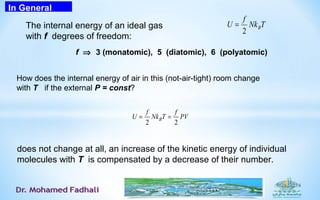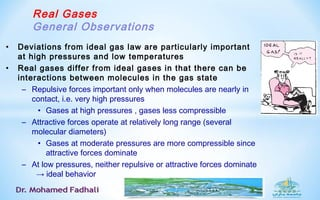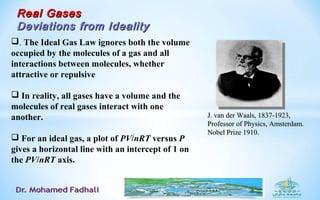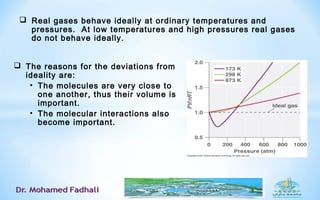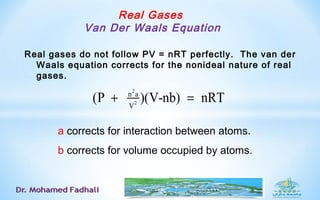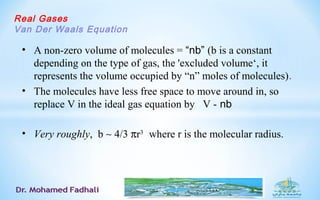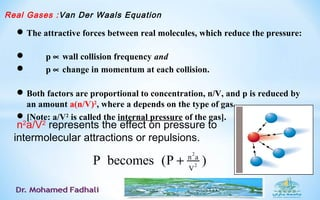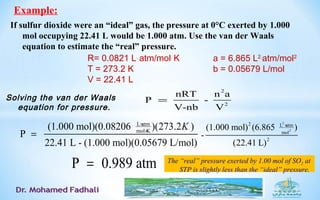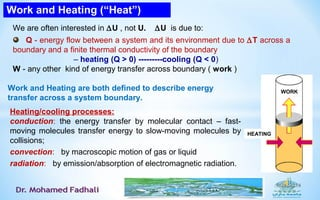1 de 19

### Thermodynamic, part 2

• 1. Ideal gases are gases of low pressure, or gases that have a low density. • We use ideal gases to approximate the more complex relationships that can exist. • Relationships between the pressure, volume and temperature are called equations of state. • These equations can be very complicated but relationship between pressure , volume and temperature can be found for ideal gases and is much simpler than the more complex general equations of state. Kinetic Theory of GasesKinetic Theory of Gases • A theory that attempts to explain the behavior of an ideal gas. •An ideal gas can be defined as: The gas that obeys the assumptions of the kinetic theory of gases.
• 2. The assumptions Kinetic Theory • Gas consists of large number of tiny particles (atoms or molecules) with freedom of movement • There exist no external forces (density constant) and no forces between particles except when they collide. • Particles, on average, separated by distances large compared to their diameters • Particles make elastic collisions with each other and with walls of container • The average kinetic energy of the gas particles is directly proportional to the Kelvin temperature of the gas
• 3. KINETIC THEORY ( ) ( ) L mv vL mvmv 2 2 collisionssuccessivebetweenTime momentumInitial-momentumFinal forceAverage − = +−− = = From Newton’s Law The internal energy of monatomic ideal gas
• 4. L mv F 2 = For a single molecule, the average force is: For N molecules, the average force is:               = L vmN F 2 3 root-mean-square speed V: is the volume Hence, The pressure is given by:
• 5. ( ) ( )2 2 1 3 22 3 1 rmsrms mvNmvNPV == 2 31 2 2KE rms Bmv k T= = We can relate the state equation of the ideal gas with its average kinetic energy as The internal energy of monatomic ideal gas 3 3 2 2BU N k T nRT= = PV BNk T⇒ = kB = R/NA & / An N N= PV nRT⇒ =But
• 6. The Equation of State of Ideal Gases P – pressure [N/m2 ] V – volume [m3 ] n – number of moles of gas T – the temperature in Kelvins [K] R – a universal constant nRTPV = Kmol J R ⋅ ≈ 31.8 The ideal gas equation of state: An equation that relates macroscopic variables (e.g., P, V, and T) for a given substance in thermodynamic equilibrium. In equilibrium (≡ no macroscopic motion), just a few macroscopic parameters are required to describe the state of a system. f (P,V,T) = 0 Geometrical representation of the equation of state: P V T an equilibrium state the equation- of-state surface R= 0.08214 L atm/mol K
• 7. Mm – Molar mass [g/mol] N – Number of molecules [molecules] NA – Avogadro’s Number of molecules per mole [molecules/mol] mM m n = AN N n = Where,TNkPV B= the Boltzmann constant kB = R/NA ≈ 1.38⋅10-23 J/K (introduced by Planck in 1899) Avogadro’s Law: equal volumes of different gases at the same P and T contain the same amount of molecules. Ideal gas, constant mass (fixed quantity of gas) 1 1 2 2 1 2 PV P V T T =
• 8. Boyle's Law (constant temperature) P = constant / V Charles Law (constant pressure) V = constant × T Gay-Lussac’s Law (constant volume) P = constant × T Important laws
• 9. Isothermals pV = constant 0 20 40 60 80 100 120 140 160 180 0.00 0.05 0.10 0.15 0.20 0.25 0.30 0.35 0.40 volume V (m 3 ) pressurep(kPa) 100 K 200 K 300 K 400 K n RT p V =
• 10. Energy in terms of Temperature At T = 300K 3 2 BKE k T= The kinetic energy is proportional to the temperature, and the Boltzmann constant kB is the coefficient of proportionality that provides one-to-one correspondence between the units of energy and temperature. If the temperature is measured in Kelvins, and the energy – in Joules: kB = 1.38×10-23 J/K 23 19 1.38 10 300 26 1.6 10 Bk T meV − − × × = ≈ ×
• 11. In General The internal energy of an ideal gas with f degrees of freedom: TNk f U B 2 = f ⇒ 3 (monatomic), 5 (diatomic), 6 (polyatomic) How does the internal energy of air in this (not-air-tight) room change with T if the external P = const? 2 2 B f f U Nk T PV= = does not change at all, an increase of the kinetic energy of individual molecules with T is compensated by a decrease of their number.
• 12. Real Gases General Observations • Deviations from ideal gas law are particularly important at high pressures and low temperatures • Real gases differ from ideal gases in that there can be interactions between molecules in the gas state – Repulsive forces important only when molecules are nearly in contact, i.e. very high pressures • Gases at high pressures , gases less compressible – Attractive forces operate at relatively long range (several molecular diameters) • Gases at moderate pressures are more compressible since attractive forces dominate – At low pressures, neither repulsive or attractive forces dominate → ideal behavior
• 13. Real GasesReal Gases Deviations from IdealityDeviations from Ideality J. van der Waals, 1837-1923,J. van der Waals, 1837-1923, Professor of Physics, Amsterdam.Professor of Physics, Amsterdam. Nobel Prize 1910.Nobel Prize 1910. . The Ideal Gas Law ignores both the volume occupied by the molecules of a gas and all interactions between molecules, whether attractive or repulsive  In reality, all gases have a volume and the molecules of real gases interact with one another.  For an ideal gas, a plot of PV/nRT versus P gives a horizontal line with an intercept of 1 on the PV/nRT axis.
• 14.  The reasons for the deviations from ideality are: • The molecules are very close to one another, thus their volume is important. • The molecular interactions also become important.  Real gases behave ideally at ordinary temperatures and pressures. At low temperatures and high pressures real gases do not behave ideally.
• 15. Real Gases Van Der Waals Equation Real gases do not follow PV = nRT perfectly. The van der Waals equation corrects for the nonideal nature of real gases. a corrects for interaction between atoms. b corrects for volume occupied by atoms. 2 2 n a V (P )(V-nb) nRT+ =
• 16. Real Gases Van Der Waals Equation • A non-zero volume of molecules = “nb” (b is a constant depending on the type of gas, the 'excluded volume‘, it represents the volume occupied by “n” moles of molecules). • The molecules have less free space to move around in, so replace V in the ideal gas equation by V - nb • Very roughly, b ∼ 4/3 πr3 where r is the molecular radius.
• 17. Real Gases :Van Der Waals Equation The attractive forces between real molecules, which reduce the pressure:  p ∝ wall collision frequency and  p ∝ change in momentum at each collision. Both factors are proportional to concentration, n/V, and p is reduced by an amount a(n/V)2 , where a depends on the type of gas. [Note: a/V2 is called the internal pressure of the gas]. 2 2 n a V P becomes (P )+ n2 a/V2 represents the effect on pressure to intermolecular attractions or repulsions.
• 18. If sulfur dioxide were an “ideal” gas, the pressure at 0°C exerted by 1.000 mol occupying 22.41 L would be 1.000 atm. Use the van der Waals equation to estimate the “real” pressure. Example: Solving the van der Waals equation for pressure. 2 2 nRT n a P - V-nb V = R= 0.0821 L. atm/mol. K T = 273.2 K V = 22.41 L a = 6.865 L2. atm/mol2 b = 0.05679 L/mol L atm mol K (1.000 mol)(0.08206 )(273.2 ) P 22.41 L - (1.000 mol)(0.05679 L/mol) K× × = 2 2 2 L atm mol 2 (1.000 mol) (6.865 ) - (22.41 L) × P 0.989 atm= The “real” pressure exerted by 1.00 mol of SO2 at STP is slightly less than the “ideal” pressure.
• 19. Work and Heating (“Heat”) We are often interested in ∆U , not U. ∆U is due to: Q - energy flow between a system and its environment due to ∆T across a boundary and a finite thermal conductivity of the boundary – heating (Q > 0) ---------cooling (Q < 0) W - any other kind of energy transfer across boundary ( work ) Heating/cooling processes: conduction: the energy transfer by molecular contact – fast- moving molecules transfer energy to slow-moving molecules by collisions; convection: by macroscopic motion of gas or liquid radiation: by emission/absorption of electromagnetic radiation. HEATING WORK Work and Heating are both defined to describe energy transfer across a system boundary.